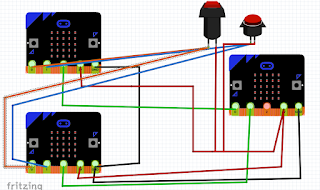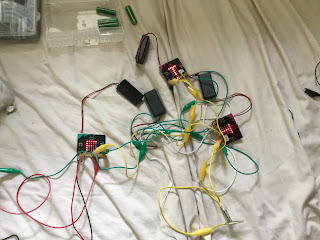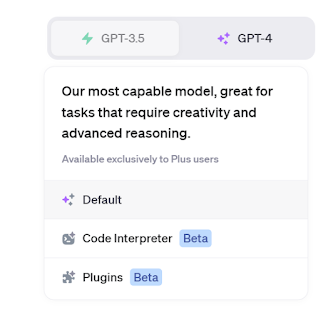## Tuesday 3 April 2018

### How to produce a Microbit neural network

This is really part two of a set of post in response to a question from Carl Simmons (@Activ8Thinking) concerning building a micro:bit simple neuron. In the previous post a single neuron was produced. This post looks at producing a network of neurons, ie. neural network; looking to solve the problem that a single neuron can't solve, making an Exclusive OR gate (XOR)

1. Quick Overview
1.1 The neuron itself

• Inputs are going to be binary
• Weighted sum is bias+W1*input1+w2*input2
• If weighted sum>=0 then the output is True (T on the LEDs) or '1'
• If weighted sum<0 then the output is False (F on the LEDs) or '0'
1.2 The XOR
Essentially for the two input case if the two inputs are different then the output is True.

The figure below shows the arrangement of the connections; pin 2 is the output of the neurons. The two micro:bits/neurons on the left of the picture taking in the two inputs, the same inputs go to these two neurons; the output from these neurons are the two inputs to the output neuron on the right.figure 1

The micro:bit objects used in Figure 1 were produced using the micro:bit Frtzing diagram available at https://github.com/microbit-foundation/dev-docs/issues/36 thanks to David Whale (@whalleygeek ) for these.

2. Neuron 1
This is the top left neurone in figure 1. This neurone is set to produce an output of TRUE (pin 2 going high) when the first input goes low and the second input goes high. The code for it is shown below.

3. Neuron 2
This is the bottom left neuron in figure 1. This neurone is set to produce an output of TRUE (pin 2 going high) when the first input goes high and the second input goes low. The code for it is shown below.

4. Output Neuron
Neuron 1
This is the right-hand neurone in figure 1. This neurone is set to produce an output of TRUE (pin 2 going high) when either inputs (outputs from neurons 1 and 2) goes high - in other words acting as an OR gate . The code for it is shown below.

The overall effect is when the two inputs to the network are high/TRUE then the output of the network (this neuron) is TRUE.

5. In Action
The wiring is messy but the effect is possible to see in these images. The top neuron is the output neuron.figure 2: inputs to the network (input 1 low and input 2 high)Figure 3: inputs to the network (input 1 high and input 2 low)figure 4: inputs to the network (both inputs the same)
6. Room for expansion
The neurons were 'trained' in this case by selecting the weights by hand, an improvement would be to get them to learn. How to do this on a micro:bit takes a bit more thinking about, but I would be interested in seeing how others solve that problem.

All opinions in this blog are the Author's and should not in any way be seen as reflecting the views of any organisation the Author has any association with. Twitter @scottturneruon

1.Very informative!!!!
robot kit

2.This comment has been removed by a blog administrator.

### ChatGPT, Data Scientist - fitting it a bit

This is a second post about using ChatGPT to do some data analysis. In the first looked at using it to some basic statistics  https://robots...